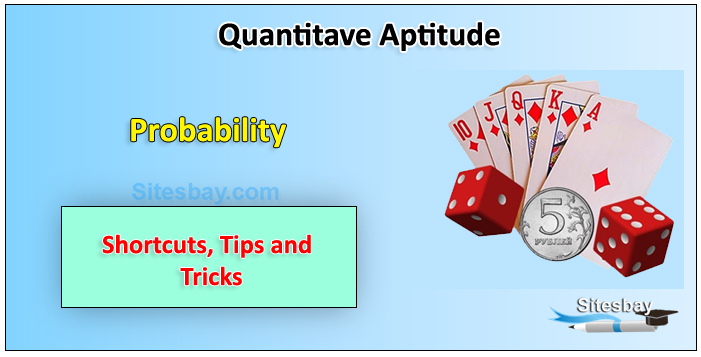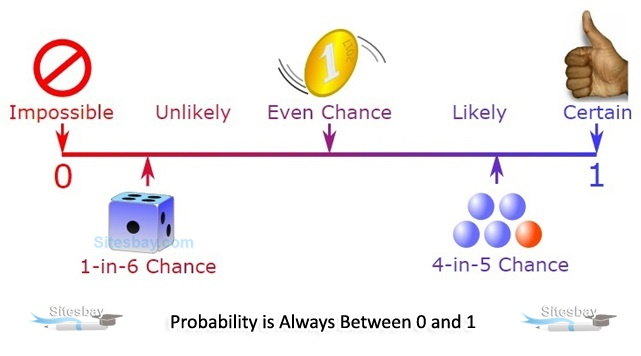# What is Probability

## Probability ProblemsProbability is a numerical description of how likely an event is to occur or how likely it is that a proposition is true. Probability is a number between 0 and 1, where, roughly speaking, 0 indicates impossibility and 1 indicates certainty.A simple example is the tossing of a fair (unbiased) coin. Since the coin is fair, the two outcomes Heads and Tails are both equally probable; the probability of "heads" equals the probability of "tails"; and since no other outcomes are possible, the probability of either Heads or Tails is 1/2 (which could also be written as 0.5 or 50%).

## Formula

 Probability of event to happen P(E) = Number of favourable outcomes Total Number of outcomes

Many events can't be predicted with total certainty. The best we can say is how likely they are to happen, using the idea of Probability

### Tossing a Coin

• Tossing a Coin

When a coin is tossed, there are two possible outcomes

• tails (T)

We say that the probability of the coin landing H is 1/2 and the probability of the coin landing T is 1/2

### Throwing Dice

• pair of dice
• Throwing Dice

When a single die is thrown, there are six possible outcomes: 1, 2, 3, 4, 5, 6. The probability of any one of them is 1/6.

## Formula

 Probability of an event happening = Number of ways it can happen Total number of outcomes